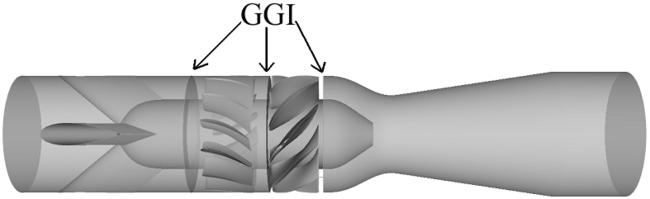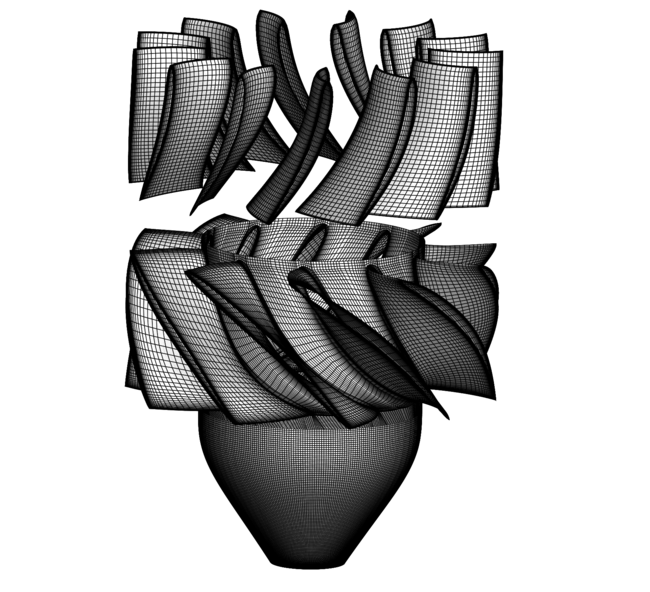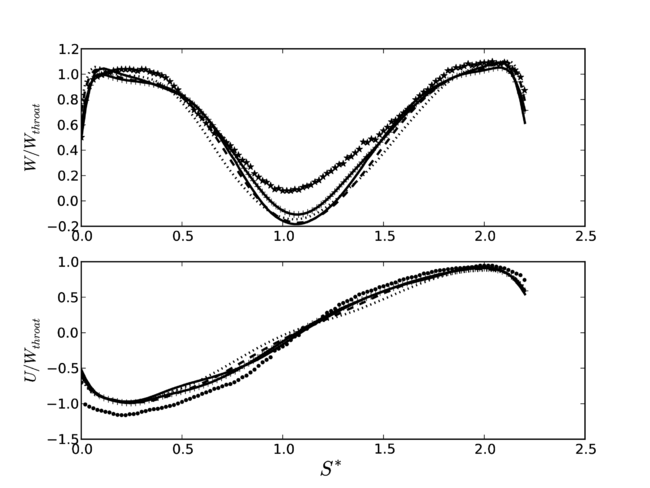# Overview of CFD Simulations

A series of numerical simulations was undertaken to study a highly swirling turbulent flow generated by rotor-stator interaction in a swirl generator . The purpose was to assess the applicability of different turbulence models in a swirling flow with a high level of unsteadiness and a significant production and dissipation of turbulence in the flow away from the wall. Nine turbulence models are compared: four high-Reynolds number models URANS, two low-Reynolds number models URANS and three hybrid URANS-LES models. These are the standard ${k-\epsilon }$, SST ${k-\omega }$, realizable ${k-\epsilon }$, RNG ${k-\epsilon }$, Launder-Sharma ${k-\epsilon }$, Lien-Cubic ${k-\epsilon }$, delayed DES Spalart-Allmaras , DDES SST ${k-\omega }$and improved DDES-SA  models. The URANS models are capable of capturing the main unsteady feature of this flow, the so-called helical vortex rope, which is formed by the strong centrifugal force and an on-axis recirculation region. However, the size of the on-axis recirculation region is overestimated by the URANS models. Although the low-Reynolds number URANS formulations resolve the boundary layers in the runner and the draft tube more accurately, they still encounter difficulties in predicting the main flow features in the adverse pressure gradient region in the draft tube. It is shown that a more detailed resolution, which is provided by the hybrid URANS-LES methods, is necessary to capture the turbulence and the coherent structures.

## SIMULATION CASE CFD

### Solution Strategy

The calculations reported herein are made using the finite-volume method in the OpenFOAM-1.6-ext CFD code. The second-order central differencing scheme is used to discretize the diffusion terms. The linear-upwind differencing is used in URANS simulations to approximate the convection term. The blended numerical scheme is used in the hybrid method. The scheme is a combination of linear-upwind differencing in the URANS region and a limited linear total variation diminishing (TVD) scheme with a conformance coefficient in the LES region. The convection term in the LES region is interpolated by 15% linear-upwind differencing and 85% central differencing. The larger the part of the central differencing in the LES region is, the smaller the time-step required. Furthermore, the second-order van Leer TVD scheme is used to approximate the convection term in the hybrid method. The numerical schemes have only small effects on the time-averaged values. Time marching is performed with an implicit second-order accurate backward differentiating scheme.

### Computational Domain

Figure 5 shows the complete computational domain. The domain is meshed in four regions in ICEM CFD using a structured multi-block approach with O–grids around the blades and in the draft tube. The strut region is included in the high-Reynolds number model simulations, but omitted in the low-Reynolds number model and hybrid URANS-LES simulations. Those simulations employ the interpolated data from the URANS simulation as inlet condition before the guide vanes.Figure 5: Computational domain and GGI applied between the four regions used by the high-Reynolds number models.

Three mesh resolutions are created, one for high-Reynolds number URANS, one for low-Reynolds number URANS, and one for hybrid URANS-LES model. Figure 6 shows the mesh for the hybrid URANS-LES simulations.Figure6: Coarse mesh used by the hybrid URANS-LES models.

The General Grid Interface (GGI) is used at the interfaces between the rotating and stationary regions, and to simplify the mesh generation of the strut region. The General Grid Interface (GGI) connections refer to the class of grid connections where the grid on either side of the two connected surfaces does not match. In general, GGI connections permit non-matching of node location, element type, surface extent, surface shape and even non-matching of the flow physics across the connection. Table 1 shows the details of the mesh resolution studies of the high-Reynolds number models and low-Reynolds number models.

Table 1: Mesh resolution study details for the high-Reynolds number and low-Reynolds number models
Mesh Strut Guide vane Runner Draft tube Total
High-Reynolds Coarse 3 × 105 7 × 105 7.6 × 105 1 × 106 2.76 × 106
High-Reynolds Fine 3 × 105 8 × 105 1.1 × 106 2.85 × 106 5.05 × 106
Low-Reynolds Coarse 9.3 × 105 1.13 × 106 1.35 × 106 3.41 × 106
Low-Reynolds Fine 9.3 × 105 1.13 × 106 2.66 × 106 4.72 × 106

The refinement is mainly done in the draft tube, which is the main area of interest in the present study. Figure \ref{meshInd} shows that the axial and tangential velocity profiles at W1 (see Fig. 1) are mesh independent for both the high-Reynolds number and low-Reynolds number model simulations.Figure 7: URANS model mesh sensitivity study. Axial (top) and tangential (bottom) velocity at W1. Solid line: RNG ${k-\epsilon }$coarse. Dashed line: RNG ${k-\epsilon }$fine. Dotted line: LS coarse. Solid line with + markers: LS fine. Star markers (*): experimental axial velocity. Point markers (${\bullet }$): experimental tangential velocity.

The survey axes, ${S*}$, at sections W0 – W2, are normalized by the throat radius, ${R_{throat}}$= 0.05m, and the velocity is normalized by the bulk velocity at the throat, ${W_{throat}}$= 3.81m/s. The survey axes, ${S*}$, begins at zero at the wall where the window is located, see Fig. 1. The coarse meshes are used for the rest of the study.

Table 2 shows the details of the mesh resolution studies of the hybrid URANS-LES turbulence models.

Table 2: Mesh resolution details for the hybrid URANS-LES models *Wall-normal spacing is equal for two meshes.
Mesh Guide vane Runner Draft tube Total ${\Delta z_{max}^{+}}$${\Delta (r\theta )_{max}^{+}}$Coarse 2.45 × 106 2.4 × 106 8.4 × 106 13.25 × 106 23.82 39.2
Fine 2.45 × 106

${y_{max}^{+}=2.2^{*}}$3.62 × 106

${y_{max}^{+}=8.1}$13.2 × 106

${y_{max}^{+}=2.5}$19.27 × 106 18.27 41.23

The main difference between the coarse and the fine mesh is again in the draft tube. The wall-parallel mesh resolution units are defined as

$\Delta z^{+}={\sqrt {{\frac {\partial w}{\partial z}}{\frac {1}{\nu }}}}\Delta z,\quad \Delta (r\theta )^{+}={\sqrt {{\frac {\partial w}{\partial (r\theta )}}{\frac {1}{\nu }}}}\Delta (r\theta ),\qquad (1)$where ${\textit {w}}$is instantaneous axial velocity, ${\nu }$is the kinematic viscosity and ${\theta }$is the tangential position. The wall-normal mesh resolution unit ${y^{+}}$is defined as

$y^{+}=u^{*}y/\nu ,\quad u^{*}=({\frac {\tau _{w}}{\rho }})^{1/2},\quad \tau _{w}=\mu ({\frac {\partial u}{\partial y}}),\qquad (2)$where ${y}$is the wall-spacing of the first cell, ${u^{*}}$is the friction velocity and ${\tau _{w}}$is the wall shear stress. The resulting values are comparable with those used before in simulations of turbulent swirling flow in a circular pipe . Therefore, the wall-parallel mesh resolution in the draft tube is sufficient for the DDES-SA model. ${y^{+}}$has a maximum value of 8.1 at the blade leading edge where there is a stagnation point. The grid spacings normalized with the throat radius, ${R_{throat}}$, in the axial, radial, and tangential directions in the draft tube are in the range of [8 × 10-4 — 0.02], [8 × 10-5 — 0.018] and [4 × 10-3 — 0.028], respectively. The wall spacings normalized with the throat radius, ${R_{throat}}$, in the runner are in the range of [2 × 10-4 — 1.16 × 10-3].

### Boundary Conditions

For the high-Reynolds number models, a constant velocity corresponding to the volume flow rate is imposed upstream of the struts. Constant values for ${k}$, ${\epsilon }$and ${\omega }$are applied at the inlet boundary, using a turbulence intensity of 10% and a viscosity ratio of ${\nu _{t}/\nu }$= 10. A series of outlet boundary conditions are studied for the pressure, and the one with the least upstream effects, the homogeneous Neumann condition, is applied at the outlet boundary. The homogeneous Neumann condition is also applied for the turbulence quantities at the outlet boundary. The inletOutlet condition, which is a homogeneous Neumann condition with blockage of a backflow, is applied at the outlet boundary for the velocity. The logarithmic law of the wall is applied at the walls in high-Reynolds number models simulations.

### Application of Physical Models

The low-Reynolds number and hybrid models have the inlet applied just upstream of the guide vanes, at the location of the first General Grid Interface (GGI) of the high-Reynolds number cases, see Fig. \ref{Comp_domain}. The inlet data is extracted from the URANS averaged results at that location, and reconstructed by spline curves where six points are used in the viscous sub-layer. The number of data points with a normalized wall distance below 10 ${(N_{y^{+}}<10)}$characterizing the viscous sublayer is often used as an indicator of the resolution of the turbulent boundary layer. This method is also verified by Gyllenram et al. , and Javadi and Nilsson [1, 9] for a highly turbulent swirling flow. To assess the sensitivity of the inlet boundary condition, Fig. \ref{fig7} shows a comparison between the Launder-Sharma ${k-\epsilon }$results using the above-mentioned boundary condition and that using constant values corresponding to the volume flow rate. The results show that the constant value and the resolution of the inlet profile has a small effect on the flow features in the draft tube. Since the extracted inlet profile yields a slightly smaller on-axis recirculation region, and closer agreement with the experimental results, that is used in the following. In the case of hybrid models, no wall-function is used.

### Computational Details

The parallel processing is done through MPI and domain decomposition. To achieve a near-optimal parallel load balancing, the computational meshes are subdivided into blocks of equal size, which are submitted to individual cores of an AMD Opteron 6220 Linux cluster. The finest mesh resolution is run on 12 nodes with 16 cores each over more than 520000 iterations (4 iterations per time-step), where each time-step requires a wall-clock time of about 75s. The time-step corresponds to 0.028 degrees of runner rotation.

The Launder-Sharma ${k-\epsilon }$simulation with the fine mesh is advanced in time with a maximum Courant-Friedrichs-Lewy (CFL) number of 2.5 and a mean CFL number of 0.03. The effect of the CFL number on the instantaneous and averaged solution of the high-Reynolds number models was examined, showing that the CFL number up to 4 has a negligible effect on the results. In the simulations using the hybrid methods, the maximum CFL number is 1.3. The maximum CFL number occurs near the nozzle, where a strong axial flow passes through a dense mesh region. The results demonstrate that little is gained by reducing the time-step and switching to a more refined numerical scheme when a relatively coarse mesh in the runner is used.

Contributed by: A. Javadia, A. Bosiocb, H Nilssona, S. Munteanc, R. Susan-Resigab — aChalmers University of Technology, Göteborg, Sweden; bUniversity Polytehnica Timişoara, Timişoara, Romania; cCenter for Advanced Research in Engineering Sciences, Romanian Academy, Timişoara Branch, Timişoara, Romania